Courses

# RD Sharma Solutions -Ex-7.4, Decimals, Class 6, Maths Class 6 Notes | EduRev

## RD Sharma Solutions for Class 6 Mathematics

Created by: Abhishek Kapoor

## Class 6 : RD Sharma Solutions -Ex-7.4, Decimals, Class 6, Maths Class 6 Notes | EduRev

The document RD Sharma Solutions -Ex-7.4, Decimals, Class 6, Maths Class 6 Notes | EduRev is a part of the Class 6 Course RD Sharma Solutions for Class 6 Mathematics.
All you need of Class 6 at this link: Class 6

Question 1

Express the following fractions as decimals:

i)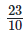Solution: 2310 = 20+310 = 2010+310 = 2+310 = 2.3

ii)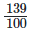Solution: 139100= 10+30+9100 = 100100+30100+9100 = 1+310+9100 = 1.39

iii)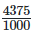Solution: 43751000 = 4000+300+70+51000 = 40001000+3001000+701000+51000 = 4+310+7100+51000 = 4.375

iv)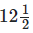Solution: 1212 = 12+12 = 12+1(52)(5) = 12+510 = 12.5

v)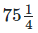Solution:7514 = 75+14 = 75+1(254)(25) = 75+25100 = 75.25

vi)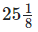Solution: 2518 = 25+1(1258)(125) = 25+1251000 = 25.125

vii)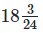Solution: 18324= 18+324 =18+18 =18+125(1125)(8) = 18+1251000 = 18.125

viii)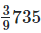Solution: 39735 = 39+735 = 39+15 = 39+1(25) (2) = 39+210 = 39.2

ix)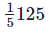Solution: 15125 =15+125 = 15+1(1)(425)(4) = 15+4100 = 15.04

1.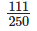111250 = 111(4250)(4) = 4441000 = 0.444

Question 2

Express the following decimals as fractions in the lowest form:

i) 0.5
Solution: =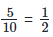ii) 2.5
Solution: =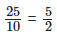iii) 0.60
Solution: =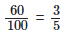iv) 0.18
Solution: =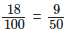v) 5.25
=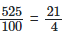vi) 7.125
=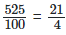vii) 15.004
Solution: =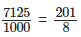viii) 20.375
Solution: =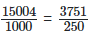ix) 600.75
Solution:=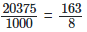Offer running on EduRev: Apply code STAYHOME200 to get INR 200 off on our premium plan EduRev Infinity!

103 docs

,

,

,

,

,

,

,

,

,

,

,

,

,

,

,

,

,

,

,

,

,

,

,

,

,

,

,

,

,

,

;